# Rigid Body Work-Precision Diagrams

##### Chris Rackauckas
using OrdinaryDiffEq, ParameterizedFunctions, ODE, ODEInterfaceDiffEq, LSODA,
Sundials, DiffEqDevTools

k(t) = 0.25*sin(t)^2

g = @ode_def RigidBody begin
dy1  = I₁*y2*y3
dy2  = I₂*y1*y3
dy3  = I₃*y1*y2 + k(t)
end I₁ I₂ I₃

p = [-2.0,1.25,-0.5]
prob = ODEProblem(g,[1.0;0.0;0.9],(0.0,10.0),p)

abstols = 1.0 ./ 10.0 .^ (6:13)
reltols = 1.0 ./ 10.0 .^ (3:10);
sol = solve(prob,Vern7(),abstol=1/10^14,reltol=1/10^14)
test_sol = TestSolution(sol)
using Plots; gr()

Plots.GRBackend()

plot(sol)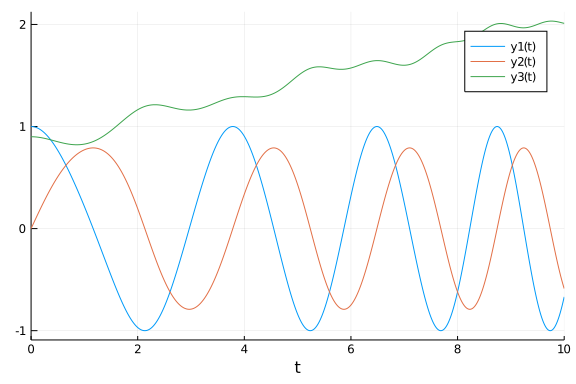setups = [Dict(:alg=>DP5())
#Dict(:alg=>ode45()) # fails
Dict(:alg=>dopri5())
Dict(:alg=>Tsit5())
Dict(:alg=>Vern6())
]
wp = WorkPrecisionSet(prob,abstols,reltols,setups;appxsol=test_sol,save_everystep=true,numruns=100,maxiters=10000)
plot(wp)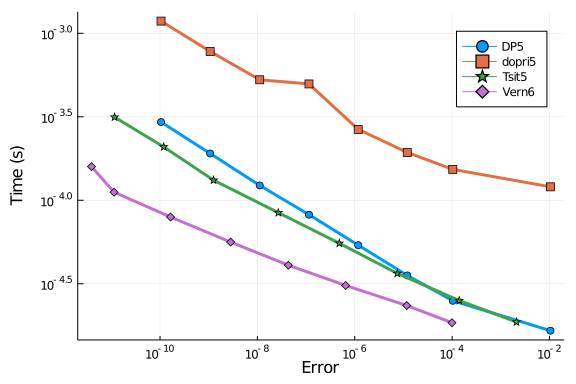The DifferentialEquations.jl algorithms once again pull ahead. This is the first benchmark we've ran where ode45 doesn't fail. However, it still doesn't do as well as Tsit5. One reason why it does so well is that the maximum norm that ODE.jl uses (as opposed to the L2 norm of Sundials, DifferentialEquations, and ODEInterface) seems to do really well on this problem. dopri5 does surprisingly bad in this test.

## Higher Order

setups = [Dict(:alg=>DP8())
#Dict(:alg=>ode78()) # fails
Dict(:alg=>Vern7())
Dict(:alg=>Vern8())
Dict(:alg=>dop853())
Dict(:alg=>Vern6())
]
wp = WorkPrecisionSet(prob,abstols,reltols,setups;appxsol=test_sol,save_everystep=false,numruns=100,maxiters=1000)
plot(wp)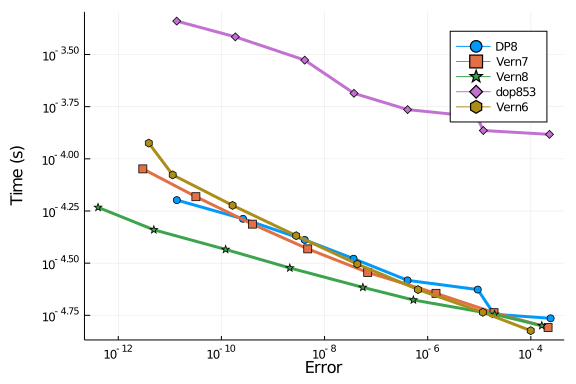setups = [Dict(:alg=>Vern7())
Dict(:alg=>Vern8())
Dict(:alg=>odex())
Dict(:alg=>lsoda())
Dict(:alg=>ddeabm())
Dict(:alg=>ARKODE(Sundials.Explicit(),order=6))
]
wp = WorkPrecisionSet(prob,abstols,reltols,setups;appxsol=test_sol,save_everystep=false,numruns=100,maxiters=1000)
plot(wp)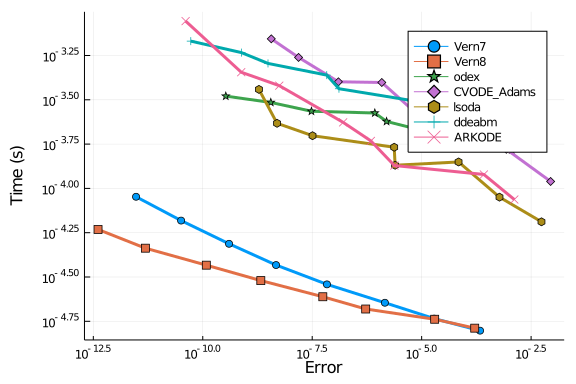## Comparison with Non-RK methods

Now let's test Tsit5 and Vern9 against parallel extrapolation methods and an Adams-Bashforth-Moulton:

setups = [Dict(:alg=>Tsit5())
Dict(:alg=>Vern9())
Dict(:alg=>VCABM())
solnames = ["Tsit5","Vern9","VCABM","AitkenNeville","Midpoint Deuflhard","Midpoint Hairer Wanner"]
wp = WorkPrecisionSet(prob,abstols,reltols,setups;appxsol=test_sol,names=solnames,
save_everystep=false,verbose=false,numruns=100)
plot(wp)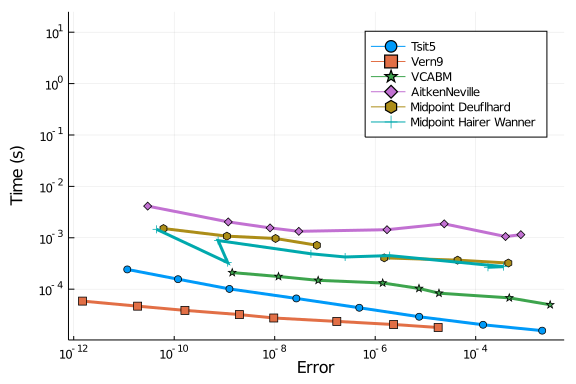setups = [Dict(:alg=>ExtrapolationMidpointDeuflhard(min_order=1, max_order=9, init_order=9, threading=false))
Dict(:alg=>ExtrapolationMidpointHairerWanner(min_order=2, max_order=11, init_order=4, sequence = :romberg, threading=true))
Dict(:alg=>ExtrapolationMidpointHairerWanner(min_order=2, max_order=11, init_order=4, sequence = :bulirsch, threading=true))]
wp = WorkPrecisionSet(prob,abstols,reltols,setups;appxsol=test_sol,names=solnames,
save_everystep=false,verbose=false,numruns=100)
plot(wp)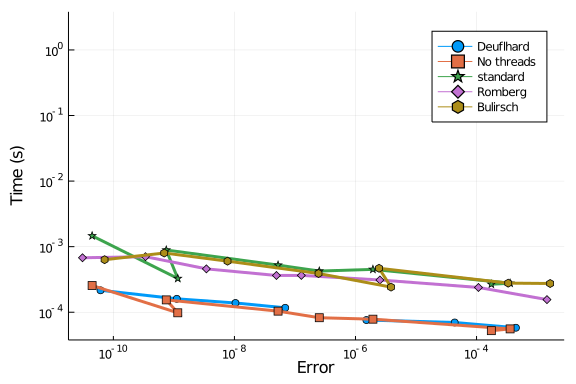setups = [Dict(:alg=>ExtrapolationMidpointHairerWanner(min_order=2, max_order=11, init_order=10, threading=true))
solnames = ["1","2","3","4","5"]
wp = WorkPrecisionSet(prob,abstols,reltols,setups;appxsol=test_sol,names=solnames,
save_everystep=false,verbose=false,numruns=100)
plot(wp)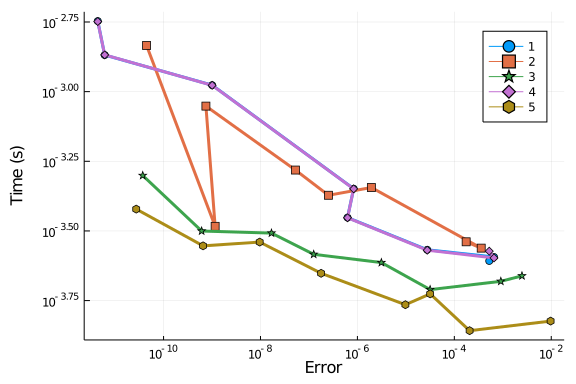### Conclusion

Once again, the OrdinaryDiffEq.jl pull far ahead in terms of speed and accuracy.

using DiffEqBenchmarks
DiffEqBenchmarks.bench_footer(WEAVE_ARGS[:folder],WEAVE_ARGS[:file])


## Appendix

These benchmarks are a part of the DiffEqBenchmarks.jl repository, found at: https://github.com/JuliaDiffEq/DiffEqBenchmarks.jl

To locally run this tutorial, do the following commands:

using DiffEqBenchmarks
DiffEqBenchmarks.weave_file("NonStiffODE","RigidBody_wpd.jmd")

Computer Information:

Jul